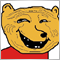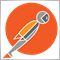# Help noob with arrays127

So I'm making my first effort into using arrays.

I'm simply trying to store close price at certain times to retrieve later in my code. Is this code going to achieve the desired result or have I ballzed up completely.

```int OnInit()
{
double EU = {iClose("EURUSD",PERIOD_H1,2), iClose("EURUSD",PERIOD_H1,8), iClose("EURUSD",PERIOD_H1,25), iClose("EURUSD",PERIOD_H1,43), iClose("EURUSD",PERIOD_H1,73), iClose("EURUSD",PERIOD_H1,121)};
double EG = {iClose("EURGBP",PERIOD_H1,2), iClose("EURGBP",PERIOD_H1,8), iClose("EURGBP",PERIOD_H1,25), iClose("EURGBP",PERIOD_H1,43), iClose("EURGBP",PERIOD_H1,73), iClose("EURGBP",PERIOD_H1,121)};
double EA = {iClose("EURAUD",PERIOD_H1,2), iClose("EURAUD",PERIOD_H1,8), iClose("EURAUD",PERIOD_H1,25), iClose("EURAUD",PERIOD_H1,43), iClose("EURAUD",PERIOD_H1,73), iClose("EURAUD",PERIOD_H1,121)};
double EN = {iClose("EURNZD",PERIOD_H1,2), iClose("EURNZD",PERIOD_H1,8), iClose("EURNZD",PERIOD_H1,25), iClose("EURNZD",PERIOD_H1,43), iClose("EURNZD",PERIOD_H1,73), iClose("EURNZD",PERIOD_H1,121)};
double EH = {iClose("EURCHF",PERIOD_H1,2), iClose("EURCHF",PERIOD_H1,8), iClose("EURCHF",PERIOD_H1,25), iClose("EURCHF",PERIOD_H1,43), iClose("EURCHF",PERIOD_H1,73), iClose("EURCHF",PERIOD_H1,121)};
double EJ = {iClose("EURJPY",PERIOD_H1,2), iClose("EURJPY",PERIOD_H1,8), iClose("EURJPY",PERIOD_H1,25), iClose("EURJPY",PERIOD_H1,43), iClose("EURJPY",PERIOD_H1,73), iClose("EURJPY",PERIOD_H1,121)};

double GU = {iClose("GBPUSD",PERIOD_H1,2), iClose("GBPUSD",PERIOD_H1,8), iClose("GBPUSD",PERIOD_H1,25), iClose("GBPUSD",PERIOD_H1,43), iClose("GBPUSD",PERIOD_H1,73), iClose("GBPUSD",PERIOD_H1,121)};
double GA = {iClose("GBPAUD",PERIOD_H1,2), iClose("GBPAUD",PERIOD_H1,8), iClose("GBPAUD",PERIOD_H1,25), iClose("GBPAUD",PERIOD_H1,43), iClose("GBPAUD",PERIOD_H1,73), iClose("GBPAUD",PERIOD_H1,121)};
double GN = {iClose("GBPNZD",PERIOD_H1,2), iClose("GBPNZD",PERIOD_H1,8), iClose("GBPNZD",PERIOD_H1,25), iClose("GBPNZD",PERIOD_H1,43), iClose("GBPNZD",PERIOD_H1,73), iClose("GBPNZD",PERIOD_H1,121)};
double GH = {iClose("GBPCHF",PERIOD_H1,2), iClose("GBPCHF",PERIOD_H1,8), iClose("GBPCHF",PERIOD_H1,25), iClose("GBPCHF",PERIOD_H1,43), iClose("GBPCHF",PERIOD_H1,73), iClose("GBPCHF",PERIOD_H1,121)};
double GJ = {iClose("GBPJPY",PERIOD_H1,2), iClose("GBPJPY",PERIOD_H1,8), iClose("GBPJPY",PERIOD_H1,25), iClose("GBPJPY",PERIOD_H1,43), iClose("GBPJPY",PERIOD_H1,73), iClose("GBPJPY",PERIOD_H1,121)};

double AU = {iClose("AUDUSD",PERIOD_H1,2), iClose("AUDUSD",PERIOD_H1,8), iClose("AUDUSD",PERIOD_H1,25), iClose("AUDUSD",PERIOD_H1,43), iClose("AUDUSD",PERIOD_H1,73), iClose("AUDUSD",PERIOD_H1,121)};
double AN = {iClose("AUDNZD",PERIOD_H1,2), iClose("AUDNZD",PERIOD_H1,8), iClose("AUDNZD",PERIOD_H1,25), iClose("AUDNZD",PERIOD_H1,43), iClose("AUDNZD",PERIOD_H1,73), iClose("AUDNZD",PERIOD_H1,121)};
double AH = {iClose("AUDCHF",PERIOD_H1,2), iClose("AUDCHF",PERIOD_H1,8), iClose("AUDCHF",PERIOD_H1,25), iClose("AUDCHF",PERIOD_H1,43), iClose("AUDCHF",PERIOD_H1,73), iClose("AUDCHF",PERIOD_H1,121)};
double AJ = {iClose("AUDJPY",PERIOD_H1,2), iClose("AUDJPY",PERIOD_H1,8), iClose("AUDJPY",PERIOD_H1,25), iClose("AUDJPY",PERIOD_H1,43), iClose("AUDJPY",PERIOD_H1,73), iClose("AUDJPY",PERIOD_H1,121)};

double NU = {iClose("NZDUSD",PERIOD_H1,2), iClose("NZDUSD",PERIOD_H1,8), iClose("NZDUSD",PERIOD_H1,25), iClose("NZDUSD",PERIOD_H1,43), iClose("NZDUSD",PERIOD_H1,73), iClose("NZDUSD",PERIOD_H1,121)};
double NH = {iClose("NZDCHF",PERIOD_H1,2), iClose("NZDCHF",PERIOD_H1,8), iClose("NZDCHF",PERIOD_H1,25), iClose("NZDCHF",PERIOD_H1,43), iClose("NZDCHF",PERIOD_H1,73), iClose("NZDCHF",PERIOD_H1,121)};
double NJ = {iClose("NZDJPY",PERIOD_H1,2), iClose("NZDJPY",PERIOD_H1,8), iClose("NZDJPY",PERIOD_H1,25), iClose("NZDJPY",PERIOD_H1,43), iClose("NZDJPY",PERIOD_H1,73), iClose("NZDJPY",PERIOD_H1,121)};

double UH = {iClose("USDCHF",PERIOD_H1,2), iClose("USDCHF",PERIOD_H1,8), iClose("USDCHF",PERIOD_H1,25), iClose("USDCHF",PERIOD_H1,43), iClose("USDCHF",PERIOD_H1,73), iClose("USDCHF",PERIOD_H1,121)};
double UJ = {iClose("USDJPY",PERIOD_H1,2), iClose("USDJPY",PERIOD_H1,8), iClose("USDJPY",PERIOD_H1,25), iClose("USDJPY",PERIOD_H1,43), iClose("USDJPY",PERIOD_H1,73), iClose("USDJPY",PERIOD_H1,121)};

double HJ = {iClose("CHFJPY",PERIOD_H1,2), iClose("CHFJPY",PERIOD_H1,8), iClose("CHFJPY",PERIOD_H1,25), iClose("CHFJPY",PERIOD_H1,43), iClose("CHFJPY",PERIOD_H1,73), iClose("CHFJPY",PERIOD_H1,121)};

return(INIT_SUCCEEDED);
}
//+------------------------------------------------------------------+
int OnTick()
{
static int currenthour;

int thishour=TimeHour(TimeCurrent());

if(currenthour!=thishour)
{
currenthour=thishour;
RunOnNewHour(thishour);
}
//+------------------------------------------------------------------+
void RunOnNewHour(int hour)
{
switch(hour)
{
case  3:
ResetSessionArrays(1,13,25,44,73,121);
break;
case  8:
ResetSessionArrays(1,12,25,42,73,121);
break;
case 15:
ResetSessionArrays(1,13,25,44,73,121);
break;
case 21:
ResetSessionArrays(1,14,25,43,73,121);
break;
}
}
//+------------------------------------------------------------------+
void ResetSessionArrays(int a, int b, int c, int d, int e, int f)
{
EU = {iClose("EURUSD",PERIOD_H1,a), iClose("EURUSD",PERIOD_H1,b), iClose("EURUSD",PERIOD_H1,c), iClose("EURUSD",PERIOD_H1,d), iClose("EURUSD",PERIOD_H1,e), iClose("EURUSD",PERIOD_H1,f)};
EG = {iClose("EURGBP",PERIOD_H1,a), iClose("EURGBP",PERIOD_H1,b), iClose("EURGBP",PERIOD_H1,c), iClose("EURGBP",PERIOD_H1,d), iClose("EURGBP",PERIOD_H1,e), iClose("EURGBP",PERIOD_H1,f)};
EA = {iClose("EURAUD",PERIOD_H1,a), iClose("EURAUD",PERIOD_H1,b), iClose("EURAUD",PERIOD_H1,c), iClose("EURAUD",PERIOD_H1,d), iClose("EURAUD",PERIOD_H1,e), iClose("EURAUD",PERIOD_H1,f)};
EN = {iClose("EURNZD",PERIOD_H1,a), iClose("EURNZD",PERIOD_H1,b), iClose("EURNZD",PERIOD_H1,c), iClose("EURNZD",PERIOD_H1,d), iClose("EURNZD",PERIOD_H1,e), iClose("EURNZD",PERIOD_H1,f)};
EH = {iClose("EURCHF",PERIOD_H1,a), iClose("EURCHF",PERIOD_H1,b), iClose("EURCHF",PERIOD_H1,c), iClose("EURCHF",PERIOD_H1,d), iClose("EURCHF",PERIOD_H1,e), iClose("EURCHF",PERIOD_H1,f)};
EJ = {iClose("EURJPY",PERIOD_H1,a), iClose("EURJPY",PERIOD_H1,b), iClose("EURJPY",PERIOD_H1,c), iClose("EURJPY",PERIOD_H1,d), iClose("EURJPY",PERIOD_H1,e), iClose("EURJPY",PERIOD_H1,f)};

GU = {iClose("GBPUSD",PERIOD_H1,a), iClose("GBPUSD",PERIOD_H1,b), iClose("GBPUSD",PERIOD_H1,c), iClose("GBPUSD",PERIOD_H1,d), iClose("GBPUSD",PERIOD_H1,e), iClose("GBPUSD",PERIOD_H1,f)};
GA = {iClose("GBPAUD",PERIOD_H1,a), iClose("GBPAUD",PERIOD_H1,b), iClose("GBPAUD",PERIOD_H1,c), iClose("GBPAUD",PERIOD_H1,d), iClose("GBPAUD",PERIOD_H1,e), iClose("GBPAUD",PERIOD_H1,f)};
GN = {iClose("GBPNZD",PERIOD_H1,a), iClose("GBPNZD",PERIOD_H1,b), iClose("GBPNZD",PERIOD_H1,c), iClose("GBPNZD",PERIOD_H1,d), iClose("GBPNZD",PERIOD_H1,e), iClose("GBPNZD",PERIOD_H1,f)};
GH = {iClose("GBPCHF",PERIOD_H1,a), iClose("GBPCHF",PERIOD_H1,b), iClose("GBPCHF",PERIOD_H1,c), iClose("GBPCHF",PERIOD_H1,d), iClose("GBPCHF",PERIOD_H1,e), iClose("GBPCHF",PERIOD_H1,f)};
GJ = {iClose("GBPJPY",PERIOD_H1,a), iClose("GBPJPY",PERIOD_H1,b), iClose("GBPJPY",PERIOD_H1,c), iClose("GBPJPY",PERIOD_H1,d), iClose("GBPJPY",PERIOD_H1,e), iClose("GBPJPY",PERIOD_H1,f)};

AU = {iClose("AUDUSD",PERIOD_H1,a), iClose("AUDUSD",PERIOD_H1,b), iClose("AUDUSD",PERIOD_H1,c), iClose("AUDUSD",PERIOD_H1,d), iClose("AUDUSD",PERIOD_H1,e), iClose("AUDUSD",PERIOD_H1,f)};
AN = {iClose("AUDNZD",PERIOD_H1,a), iClose("AUDNZD",PERIOD_H1,b), iClose("AUDNZD",PERIOD_H1,c), iClose("AUDNZD",PERIOD_H1,d), iClose("AUDNZD",PERIOD_H1,e), iClose("AUDNZD",PERIOD_H1,f)};
AH = {iClose("AUDCHF",PERIOD_H1,a), iClose("AUDCHF",PERIOD_H1,b), iClose("AUDCHF",PERIOD_H1,c), iClose("AUDCHF",PERIOD_H1,d), iClose("AUDCHF",PERIOD_H1,e), iClose("AUDCHF",PERIOD_H1,f)};
AJ = {iClose("AUDJPY",PERIOD_H1,a), iClose("AUDJPY",PERIOD_H1,b), iClose("AUDJPY",PERIOD_H1,c), iClose("AUDJPY",PERIOD_H1,d), iClose("AUDJPY",PERIOD_H1,e), iClose("AUDJPY",PERIOD_H1,f)};

NU = {iClose("NZDUSD",PERIOD_H1,a), iClose("NZDUSD",PERIOD_H1,b), iClose("NZDUSD",PERIOD_H1,c), iClose("NZDUSD",PERIOD_H1,d), iClose("NZDUSD",PERIOD_H1,e), iClose("NZDUSD",PERIOD_H1,f)};
NH = {iClose("NZDCHF",PERIOD_H1,a), iClose("NZDCHF",PERIOD_H1,b), iClose("NZDCHF",PERIOD_H1,c), iClose("NZDCHF",PERIOD_H1,d), iClose("NZDCHF",PERIOD_H1,e), iClose("NZDCHF",PERIOD_H1,f)};
NJ = {iClose("NZDJPY",PERIOD_H1,a), iClose("NZDJPY",PERIOD_H1,b), iClose("NZDJPY",PERIOD_H1,c), iClose("NZDJPY",PERIOD_H1,d), iClose("NZDJPY",PERIOD_H1,e), iClose("NZDJPY",PERIOD_H1,f)};

UH = {iClose("USDCHF",PERIOD_H1,a), iClose("USDCHF",PERIOD_H1,b), iClose("USDCHF",PERIOD_H1,c), iClose("USDCHF",PERIOD_H1,d), iClose("USDCHF",PERIOD_H1,e), iClose("USDCHF",PERIOD_H1,f)};
UJ = {iClose("USDJPY",PERIOD_H1,a), iClose("USDJPY",PERIOD_H1,b), iClose("USDJPY",PERIOD_H1,c), iClose("USDJPY",PERIOD_H1,d), iClose("USDJPY",PERIOD_H1,e), iClose("USDJPY",PERIOD_H1,f)};

HJ = {iClose("CHFJPY",PERIOD_H1,a), iClose("CHFJPY",PERIOD_H1,b), iClose("CHFJPY",PERIOD_H1,c), iClose("CHFJPY",PERIOD_H1,d), iClose("CHFJPY",PERIOD_H1,e), iClose("CHFJPY",PERIOD_H1,f)};
}
//+------------------------------------------------------------------+
```1079

You should probably declare the arrays as global without values and then copy one by one the values in OnInit or OnTick, EG = iClose("EURGBP",PERIOD_H1,2); EG = iClose("EURGBP",PERIOD_H1,8); and so on.

Also things declared in OnInit won't be available in OnTick.20228

1. Arr[i] = aValue; not arr[n] = { value, value, value...};
2. type Arr[n] = { const1, const2, ...}; or simply type Arr = { const1, const2, ...};  can not use non-constant values to initialize a new array.

3. Don't try to use any price or server related functions in OnInit (or on load,) as there may be no connection/chart yet:
1. Terminal starts.
2. Indicators/EAs are loaded. Static and globally declared variables are initialized. (Do not depend on a specific order.)
3. OnInit is called.
4. For indicators OnCalculate is called with any existing history.
5. Human may have to enter password, connection to server begins.
6. New history is received, OnCalculate called again.
7. New tick is received, OnCalculate/OnTick is called. Now TickValue, TimeCurrent and prices are valid.127

Thanks all. I'll work on a replacement code and display for comment2392

Robert Browne:
Thanks all. I'll work on a replacement code and display for comment

DRY :)